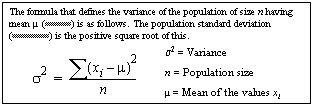# var_pop

Computes the statistical variance of a population consisting of a numeric expression, as a double. varp is an alias for var_pop, and uses the same syntax.

## Syntax

`var_pop ( [all | distinct] expression )`

## Parameters

• all – applies var_pop to all values. all is the default.
• distinct – eliminates duplicate values before var_pop is applied.
• expression – is an expression—commonly a column name—in which its population-based variance is calculated over a set of rows.

## Examples

• Example 1 – Lists the average and variance of the advances for each type of book in the pubs2 database:
```select type, avg(advance) as "avg", var_pop(advance)
as "variance" from titles group by type order by type```

## Usage

Computes the population variance of the provided value expression evaluated for each row of the group (if distinct was specified, then each row that remains after duplicates have been eliminated), defined as the sum of squares of the difference of value expression, from the mean of value expression, divided by the number of rows in the group or partition.

Formula for Population-Related Statistical Aggregate FunctionsFor general information about aggregate functions, see Aggregate Functions in Transact-SQL Users Guide.

## Standards

ANSI SQL – Compliance level: Transact-SQL extension.

## Permissions

Any user can execute var_pop.

Related reference
stddev_pop
stddev_samp
var_samp